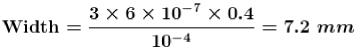Courses

# Inteference NAT Level - 1

## 10 Questions MCQ Test Topic wise Tests for IIT JAM Physics | Inteference NAT Level - 1

Description
This mock test of Inteference NAT Level - 1 for Physics helps you for every Physics entrance exam. This contains 10 Multiple Choice Questions for Physics Inteference NAT Level - 1 (mcq) to study with solutions a complete question bank. The solved questions answers in this Inteference NAT Level - 1 quiz give you a good mix of easy questions and tough questions. Physics students definitely take this Inteference NAT Level - 1 exercise for a better result in the exam. You can find other Inteference NAT Level - 1 extra questions, long questions & short questions for Physics on EduRev as well by searching above.
*Answer can only contain numeric values
QUESTION: 1

### Two slits separated by a distance of 1 mm, are illuminated with red light of wavelength 6.5 x 10-7 m. The interference fringes are observed on a screen placed 1 m from the slits. Find the distance (in mm) between the third dark fringe and the fifth bright fringe on the same side of the central maxima.

Solution: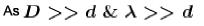Hence we can use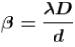So distance between 5th bright fringe and 3rd dark fringe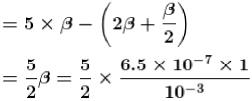= 1.625 mm

*Answer can only contain numeric values
QUESTION: 2

### The path difference between two interfering waves at a point on the screen is λ/6. The ratio of intensity at this point and that at the central bright fringe will be : (Assume that intensity due to each slit in same)

Solution:

At path difference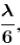phase difference is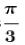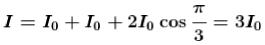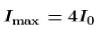So the required ratio is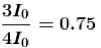*Answer can only contain numeric values
QUESTION: 3

### If the distance between the first maxima and fifth minima of a double slit pattern is 7 mm and the slits are separated by 0.15 mm with the screen 50 cm from the slits, then wavelength (in nm) of the light used is :

Solution:

There are three and a half fringes from first maxima to fifth minima as shown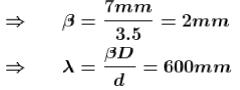*Answer can only contain numeric values
QUESTION: 4

Calculate the width (in mm) of central maxima. If light of 9000 A incidents upon a slit of width 1.5 mm. Screen is kept 150 cm away from the slit.

Solution:

Width of central maxima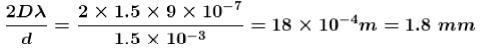*Answer can only contain numeric values
QUESTION: 5

If the ratio of the intensity of two coherent sources is 4, then the visibility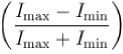of the fringes is.

Solution: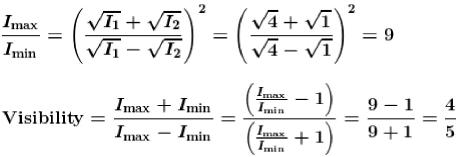*Answer can only contain numeric values
QUESTION: 6

If the relative permitivity and relative permeability of a medium are 2 & 1.28 respectively, then refractive index of the material is.

Solution:

Refractive Index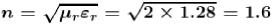*Answer can only contain numeric values
QUESTION: 7

Two lightwaves are given by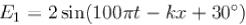and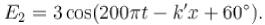The ratio intensity of first wave to that of second wave is.

Solution: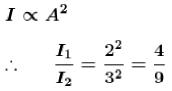*Answer can only contain numeric values
QUESTION: 8

A light ray incidents on water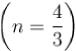surface from air and reflected part of it is found to be polarized. Find the deviation (in degrees) of refracted light from its original path :

Solution: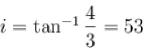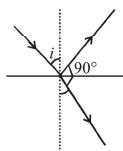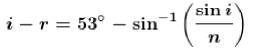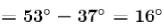*Answer can only contain numeric values
QUESTION: 9

In young’s double slit experiment, we get 60 fringes in the field of view of monochromatic light of wavelength 4000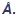. If we use monochromatic light of wavelength 6000, then the number of fringes that would be obtained in the same field of view is.

Solution:

λ α (Fringe width)
Higher 'λ' means wider fringes.
At higher wavelength, same space on the screen is occupied by wider fringes, and hence lesser number of fringes.
Also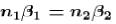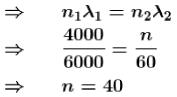*Answer can only contain numeric values
QUESTION: 10

A single slits of width 0.1 mm is illuminated by parallel light of wavelength 6000and diffraction bands are observed on a screen 40 cm from the slit. The distance (in mm) of third dark band from the central bright band is :

Solution: